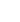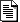# Index of /~mate/miscNameLast modifiedSizeDescriptionParent Directory   -arcsec_substitution.pdf2017-03-08 21:01 108Kcardinalities.pdf 2020-01-30 13:36 109Kcayley_hamilton.pdf 2017-11-07 14:59 171Kcharacter_lin_partdi..>2011-10-06 12:02 122Kcharpits_method_comp..>2011-12-14 14:17 136Kcompl.pdf 2015-11-22 14:06 66Kcyclic_decomposition..>2017-12-05 14:50 331Kdeterminants.pdf 2017-10-01 17:07 102Kechelon.pdf 2017-02-08 15:07 56Keuclidean_alg.pdf 2017-09-12 21:54 63Keuclids_lemma.pdf 2018-03-14 22:43 37Kexp_x.pdf 2019-02-06 08:55 118Kfirst_integrals_part..>2011-12-11 21:22 64Kfirst_ord_partdiff_e..>2011-12-11 21:24 140Kfrenet_serret.pdf 2018-09-01 15:51 107Kgreatest_mistake.html 2014-09-08 22:46 1.2Kheartofmath/ 2011-02-20 15:22 -hyperbolic_functions..>2020-11-30 12:40 92Kinner_product_spaces..>2019-11-27 22:21 241Kjordan_canonical.pdf 2017-09-14 20:18 251Klaplacian_polarcoord..>2015-01-15 16:08 104Klogic.pdf 2017-05-24 21:58 83Kmixedpartial.pdf 2017-02-23 17:49 83Kpigeonhole.pdf 2014-04-30 17:04 51Krotation.pdf 2021-05-04 17:40 87Krowspace.pdf 2017-02-26 18:01 71Ksinxperx.pdf 2019-02-06 08:10 51Ksqrt.pdf 2015-09-24 23:00 46Ktaylor.pdf 2015-04-26 08:37 63Ktelescoping_sums.pdf 2019-11-25 17:07 80Ktime_series.pdf 2019-11-04 17:45 1.4Mtotally_bded.pdf 2014-09-05 15:15 71Kunique_factorization..>2018-02-21 18:04 34K

Miscellaneous notes

# Miscellaneous notes

Most of these notes were written as supplementary material for courses I have taught.

## Analysis

compl.pdf has the title "Axiom of completeness," and it discusses the completeness of the real line. This is part of a larger set of notes ../anl/analysis.pdf on elementary real analysis, discussing convergence theorems, differentiation, and Riemann integration, entitled "Supplementary notes on introduction to analysis."
exp_x.pdf has the title "The natural exponential function," and it discusses an approach to the function exp(x) that does not rely on integration.
hyperbolic_functions.pdf has the tile "Hyperbolic functions," and it contains an introduction to hyperbolic functions and their relationship to trigonometric functions. When doing so, it discusses exponentiation with imaginary exponents that may have more intuitive appeal than the standard discussion involving Taylor series.
laplacian_polarcoord_higherdim.pdf is a set of notes entitled "The Laplace operator in polar coordinates in several dimensions." It discusses the analogs of polar and spherical coordinates in higher dimensions, and discusses the Laplace operator in these coordinates.
mixedpartial.pdf is a set of notes with the title "On the equality of mixed partial derivatives" that describes Peano's example where to mixed partial derivatives are not equal, and then discusses a number of conditions, some less well known, ensuring the equality of mixed partial derivatives.
sinxperx.pdf discusses the limit of sin t/t at t=0.
taylor.pdf has the title "The remainder term in Taylor's formula"; it discusses various forms of the remainder term of the Taylor formula. This is part of a larger set of notes ../anl/analysis.pdf on elementary real analysis just mentioned.
totally_bded.pdf is a set of notes entitled "Totally bounded spaces," and it shows that a metric space is compact if and only if it is totally bounded and complete.

## Linear Algebra

cayley_hamilton.pdf is a note with title "The Cayley--Hamilton Theorem" proves Cayley--Hamilton Theorem using the adjugate (classic adjoint) of a matrix. It includes a short discussion of formal polynomials and the evaluation operator.
cyclic_decomposition.pdf is a note with title "The cyclic decomposition theorem" proves the cyclic decomposition theorem for a vector space over an arbitrary field, and discusses some consequences, including the Jordan canonical form of a matrix, and the square root of a positive matrix.
echelon.pdf is a note entitled "The uniqueness of the row echelon form" shows that the row echelon form of a matrix is unique.
jordan_canonical.pdf is a note with title "The Jordan canonical form" proves the existence of the Jordan canonical form of a matrix over an algebraically closed field. It gives an example finding the minimal polynomial of a matrix, and uses it to find its Jordan canonical form.
rotation.pdf is a note entitled "The rotation of a coordinate system as a linear transformation" uses the theory of linear transformations to discuss rotations of the coordinate system in the plane.
rowspace.pdf is a note with title "The row space of a matrix" shows that two matrices with the same rowspace have the same row echelon form, implying the uniqueness of the row echelon form.
determinants.pdf is a note entitled "Determinants." and it gives an introduction to the theory of determinants. This is part of a larger set of notes ../nml/numanal.pdf with the title "Introduction to numerical analysis with C programs," intended as a complete set of notes for a one-semester course in numerical analysis.

## Partial differential equations

character_lin_partdiff_eq.pdf has the title "characteristic manifolds of linear partial differential equations" discusses the Cauchy problem for higher order linear partial differential equations, and describes the significance of the characteristic manifold (not to be confused with characteristic curves used in solving first order partial differential equations).
first_integrals_partdiff_eq.pdf is a note with title "The first integral of a homogeneous linear partial differential equation," and it discusses some simple examples to find the first integral.
first_ord_partdiff_eq.pdf has the title "First order partial differential equations," and discusses the basic theory of first order partial differential equations, describes characteristic curves and the complete integral, with some examples.
charpits_method_compl_int.pdf entitled "Charpit's method to find the complete integral" describes a method to find the complete integral of a first order partial differential equation of two independent variables.

## Time Series

time_series.pdf is a set of notes entitled "Aspect of time series," and it discusses some aspects, mainly mathematical, of time series analysis. It is written as a notes for a course on time series to accompany in introductory textbook on the subject.

## Other

cardinalities.pdf is a set of notes entitled "Cardinalities," an elementary discussion of countably infinite and continuum cardinalities. It also includes a discussion of the Cantor-Schröder-Bernstein theorem saying that if each of two sets can be mapped into the other in a one-to-one way then the to sets can also be mapped onto each other in a one-to-one way. The proof described in two different ways, one is the standard way, the other is a different way with intuitive appeal.
euclidean_alg.pdf is a short note describing the Euclidean algorithm to calculate the greatest common divisor of two numbers and to represent this greatest common divisor as a linear combination of the two numbers. This is part of a larger set of notes entitled "Introduction to open computing" to be found at ../open_computing/open.pdf . The notes discuss Unix and Pascal on an elementary level. These notes have not been updated for a while, and a large part of it is now obsolete, so it can still be used as an elementary introduction to using Unix or Linux.
logic.pdf discusses basic concepts of propositional and first order predicate logic. It is part of the notes ../anl/analysis.pdf mentioned above.
pigeonhole.pdf is a short note entitled "Approximation of numbers by fractions" gives an application of the pigeon-hole principle to show that every real number can be approximated with a fraction such that the goodness of the approximation is quadratic in terms of the denominator of the fraction.
sqrt.pdf is a short note with the title "Irrationality of square roots" showing that the square root of a positive integer is either an integer or it is irrational. The proof does not rely on the standard argument using divisibility by a prime occurring with an odd exponent in the prime factorization of the number, and it was apparently first described in the twentieth century.
telescoping_sums.pdf is a short note discussing some notable telescoping sums involving products of consecutive integers or the reciprocals of products of consecutive integers.
greatest_mistake.html is a link to a writing called "The greatest math mistake of the century," meaning the twentieth century, is a humorous story involving Bertrand Russel, Alfred North Whitehead, David Hilbert, Kurt Gödel, Alan Turing, and John von Neumann leading to the invention of the von Neumann computer architecture, the standard architecture of modern computers. The author of the story is unknown to me; it was posted for many years on the Internet, then disappeared from one location and appeared at another. I rescued the writing and I post it now before it lost is forever. I welcome any information about the origin of these notes.
The directory heartofmath/ contains a problem set with and without solutions as problems.pdf and solutions.pdf related to some material in the book Edward B. Burger and Michael Starbird, The Heart of Mathematics: An Invitation to Effective Thinking, Wiley, various editions, that I used in a basic mathematics course.

Last updated: Sun Dec 18 14:48:04 EST 2016.